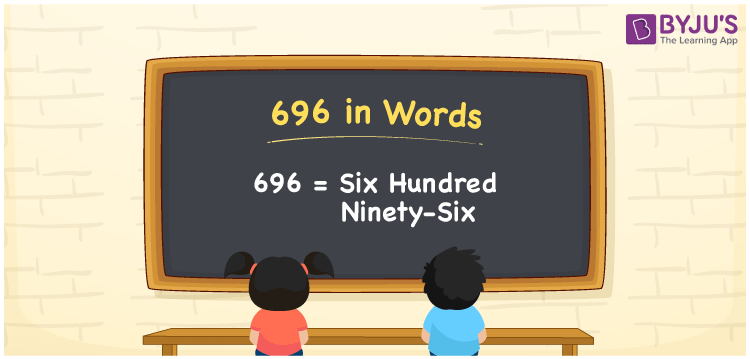# 696 in Words

696 in words is written as Six hundred ninety-six. In both the International System of Numerals and the Indian System of Numerals, 696 is written as Six hundred ninety-six. The number 696 is a Cardinal Number as it defines some quantity. For example, “there is a shortage of 696 rupees”.

 696 in Words Six hundred ninety-six Six hundred ninety-six in Number 696

## 696 in English Words

We write 696 in English Words using the letters of English alphabets. Therefore, we read 696 in English as “Six hundred ninety-six”## How to Write 696 in Words?

To write 696 in words, we shall use the place value chart. In the place value chart, write 6 in the hundreds, 9 in the tens, and 6 in the ones, respectively. Now let us make a place value chart to write the number 696 in words.

 Hundreds Tens Ones 6 9 6

Thus, we can write the expanded form as

6 × Hundred + 9 × Ten + 6 × One

= 6 × 100 + 9 × 10 + 6 × 1

= 600 + 90 + 6

= 696

= Six hundred ninety-six.

696 is a natural number, the successor of 695 and the predecessor of 697.

696 in words – Six hundred ninety-six

• Is 696 an odd number? – No
• Is 696 an even number? – Yes
• Is 696 a perfect square number? – No
• Is 696 a perfect cube number? – No
• Is 696 a prime number? – No
• Is 696 a composite number? – Yes

## Frequently Asked Questions on 696 in Words

Q1

### How to write 696 in words?

696 in words is written as Six hundred ninety-six.
Q2

### How to write 696 in words in the International and Indian System of Numerals?

In both the system of numerals, 696 is written as Six hundred ninety-six.
Q3

### What is the successor of 696?

The successor of 696 is 697.